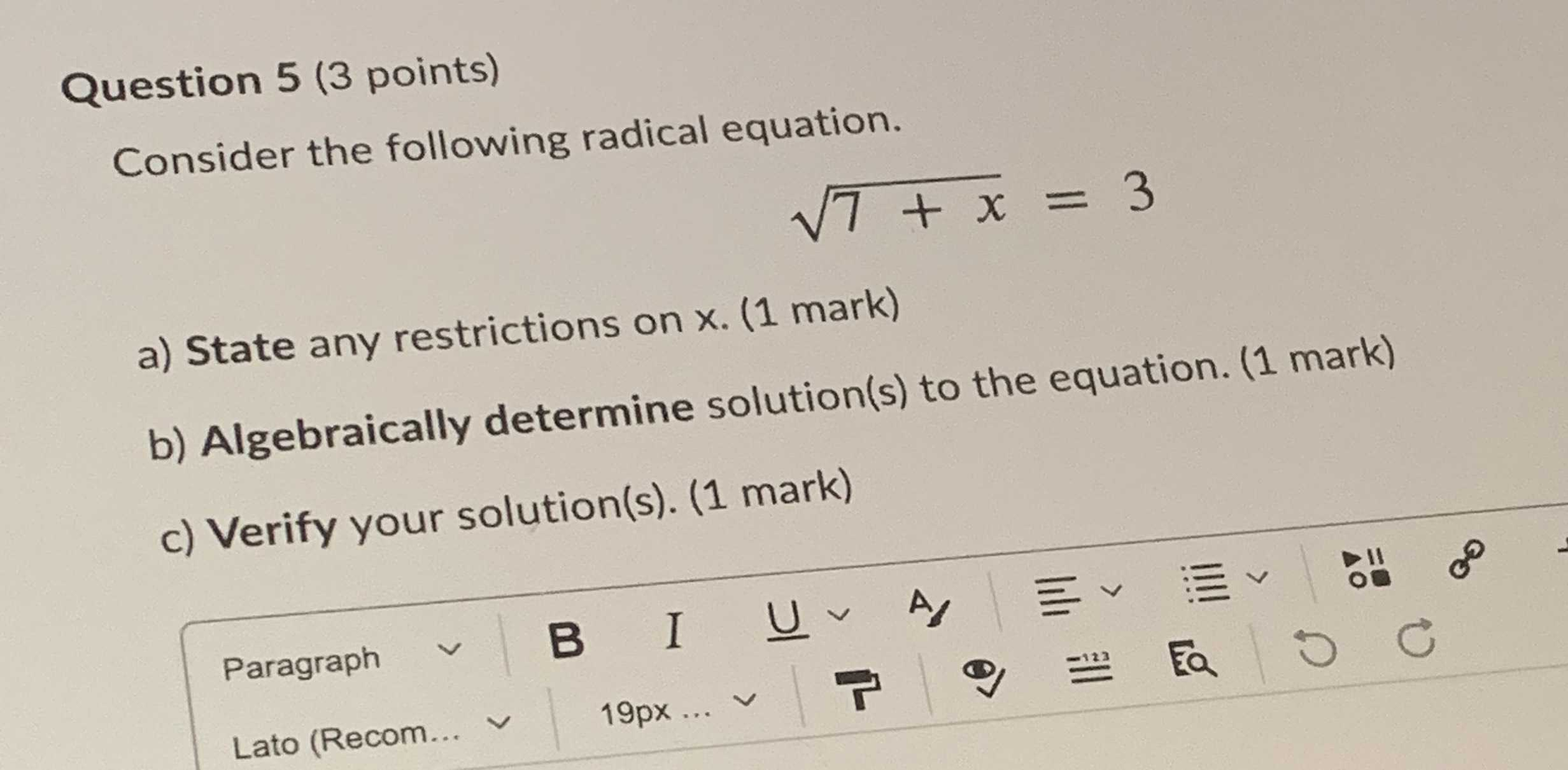### ¿Todavía tienes preguntas de matemáticas?

Pregunte a nuestros tutores expertos
Algebra
PreguntaConsider the following radical equation. $$\sqrt { 7 + x } = 3$$

a) State any restrictions on $$x$$ .

b) Algebraically determine solution(s) to the equation.

a) x > -7  , b) 7 + x = 9  , x = 2  , c) $$\sqrt{7+ 2} = 3$$